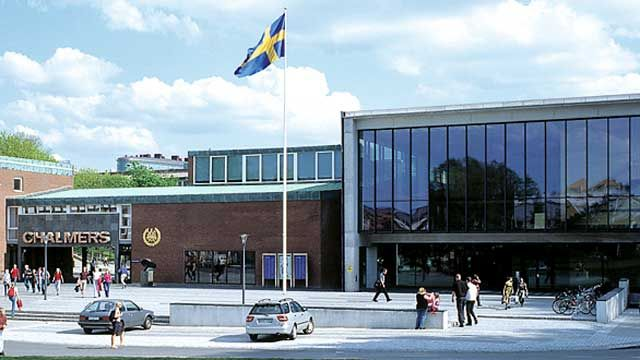# Chalmers University of Technology Integrates MATLAB Throughout Core Mathematics Curriculum

## Challenge

Teach mathematics and mathematical modeling to students across multiple disciplines and two universities, and prepare them to meet the needs of industry

## Solution

Integrate MATLAB throughout the core mathematics curriculum and provide interdisciplinary exercises in practical problem-solving

## Results

• Interdepartmental collaboration improved
• Students taught skills required by industry
• Students self-identified as expert users

“We train our students to use MATLAB as an interactive tool not only for completing the assigned exercises but also for investigating mathematical problems and having fun. We are increasing our focus on interdisciplinary programs, and MATLAB is perfect for integrating mathematics with other subjects.”

Dr. Tommy Gustafsson, Chalmers University of TechnologyChalmers University in Gothenburg, Sweden.

Chalmers University of Technology and the University of Gothenburg share a mathematical sciences department that offers courses to students at both universities. The department’s undergraduate curriculum includes a set of core courses for students in engineering, science, and math programs. Through these courses, almost 1000 students each year learn how to use MATLAB® to solve problems relevant to their area of study. The faculty adopted this approach to engage students in learning foundational math concepts and to prepare them for upper-level courses and careers that require skills in mathematical modeling.

“MATLAB is the common thread running through all our core math courses,” says Dr. Tommy Gustafsson, director of studies for mathematics at Chalmers. “MATLAB serves as a bridge between math and other subjects—such as chemistry or architecture—that helps students connect with the course material and understand how it is applied in industry.”

## Challenge

In the past, students in the early mathematics courses were given relatively simple assignments on concepts taught in lectures. The department’s faculty soon recognized two shortcomings of this approach. First, the problems were not difficult enough to engage the students. Second, they were abstract and not directly relevant to practical engineering or scientific challenges. “Instead of school problems, as I called them, we wanted to present students with real problems, to help them understand and apply what they learned,” says Gustafsson. “Many industries in Sweden need people who understand mathematical modeling, and we wanted to prepare our students to meet that demand.”

The department also wanted to provide students at Chalmers and the University of Gothenburg with easy access to the tools they needed to complete assignments and projects in an interdisciplinary curriculum.

## Solution

The mathematical sciences department faculty integrated MATLAB throughout core and upper-level courses, and both universities acquired a Campus-Wide License, enabling students to access MATLAB on either campus.

Instructors have tailored the first- and second-year courses to students in chemistry and bioengineering programs, as well as to students in architecture and technical programs.

Chemical and bioengineering students begin with an introduction to MATLAB, including built-in functions and plotting. They then learn basic programming structures in MATLAB, and complete an assignment in which they program Conway’s Game of Life. The final two assignments in this first course explore bisection, linearization, and Newton’s method.

The second course covers the application of integrals and ordinary differential equations in MATLAB. The students use MATLAB to model reaction kinetics and the oxidation of NO to NO2.

In the third and fourth courses, students solve linear systems of differential equations. Students write their own solvers before using the built-in MATLAB solvers. Using MATLAB, students reproduce a plot from a chemistry textbook showing the probability of finding the electron in a certain area around a hydrogen atom.

First-year students in architecture and technology programs also start with an introduction to MATLAB, covering integrals, differential equations, and numeric computation. With MATLAB, they complete assignments using calculus in one variable and multivariate calculus.

Students in the Shape, Color, and Architectural Tools course explore geometries used in architecture and engineering. In a follow-up course on linear algebra, students complete a final project in which they use MATLAB to model the glass roof over the courtyard of the British Museum.

Students at all levels—including graduates—continue to use MATLAB throughout their studies for course assignments, individual and team projects, and student engineering competitions.

## Results

• Interdepartmental collaboration improved. “MATLAB has enabled the mathematical sciences department to improve its collaboration with other departments, which helps students connect to the subject they are studying,” says Dr. Gustafsson. “Chemistry students solve math problems related to chemical processes, and architectural students apply mathematical modeling to bridges, buildings, and other structures—all in MATLAB.”

• Students taught skills required by industry. “Our industrial partners in Sweden and Europe want students who know how to create and use mathematical models in MATLAB,” says Dr. Gustafsson. “We used MATLAB extensively when I worked in the automotive industry. Our students use it in much the same way as they learn to solve real problems.”

• Students self-identified as expert users. In a survey of 115 teams in the Formula Student undergraduate engineering competition, the team from Chalmers and University of Gothenburg was one of just four teams who rated themselves as expert MATLAB users. “I was not surprised by this result, because MATLAB is deeply integrated into all teaching in our department,” says Dr. Gustafsson.